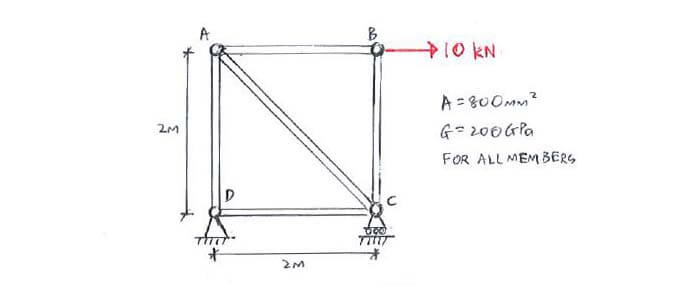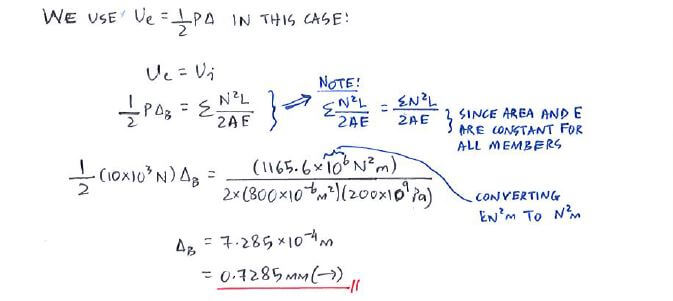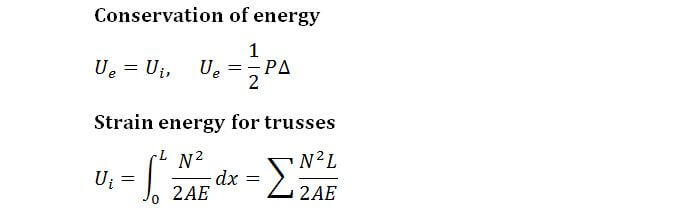# Example QuestionClick below to show answer. X

#### Step 3: Ue = Ui to calculate ΔB (→) Step 3: Ue = Ui to calculate ΔB (→)#### Useful formula: Useful formula:Now that you've mastered this question, you can try Question 1.